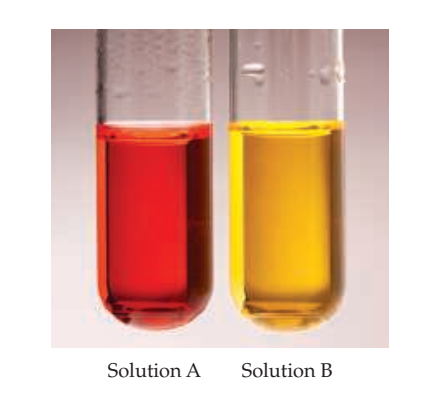×
Get Full Access to Chemistry: The Central Science - 14 Edition - Chapter 16 - Problem 16.3
Get Full Access to Chemistry: The Central Science - 14 Edition - Chapter 16 - Problem 16.3

×

# ?The indicator methyl orange has been added to both of the following solutions. Based on the colors, classify each statement as true or false:ISBN: 9780134414232 1274

## Solution for problem 16.3 Chapter 16

Chemistry: The Central Science | 14th Edition

• Textbook Solutions
• 2901 Step-by-step solutions solved by professors and subject experts
• Get 24/7 help from StudySoup virtual teaching assistantsChemistry: The Central Science | 14th Edition

4 5 1 267 Reviews
16
0
Problem 16.3

The indicator methyl orange has been added to both of the following solutions. Based on the colors, classify each statement as true or false:

(a) The pH of solution A is definitely less than 7.00.

(b) The pH of solution B is definitely greater than 7.00.

(c) The pH of solution B is greater than that of solution A. [Section 16.4]Step-by-Step Solution:

Step 1 of 5) Now let’s consider another example, the expansion of an ideal gas at constant temperature (referred to as an isothermal process). In the cylinder-piston arrangement of Figure 19.4, when the partition is removed, the gas expands spontaneously to fill the evacuated space. Can we determine whether this particular isothermal expansion is reversible or irreversible Because the gas expands into a vacuum with no external pressure, it does no P9V work on the surroundings. (Section 5.3) Thus, for the expansion, w = 0. We can use the piston to compress the gas back to its original state, but doing so requires that the surroundings do work on the system, meaning that w 7 0 for the compression. In other words, the path that restores the system to its original state requires a different value of w (and, by the first law, a different value of q) than the path by which the system was first changed. The fact that the same path cannot be followed to restore the system to its original state indicates that the process is irreversible.

Step 2 of 2

##### ISBN: 9780134414232

This full solution covers the following key subjects: . This expansive textbook survival guide covers 29 chapters, and 2820 solutions. Chemistry: The Central Science was written by and is associated to the ISBN: 9780134414232. This textbook survival guide was created for the textbook: Chemistry: The Central Science, edition: 14. The answer to “?The indicator methyl orange has been added to both of the following solutions. Based on the colors, classify each statement as true or false: (a) The pH of solution A is definitely less than 7.00. (b) The pH of solution B is definitely greater than 7.00. (c) The pH of solution B is greater than that of solution A. [Section 16.4]” is broken down into a number of easy to follow steps, and 61 words. The full step-by-step solution to problem: 16.3 from chapter: 16 was answered by , our top Chemistry solution expert on 10/03/18, 06:29PM. Since the solution to 16.3 from 16 chapter was answered, more than 210 students have viewed the full step-by-step answer.

## Discover and learn what students are asking

Calculus: Early Transcendental Functions : Exponential and Logarithmic Functions
?Evaluating an Expression In Exercises 1 and 2, evaluate the expressions. (a) $$25^{3 / 2}$$ (b) $$81^{1 / 2}$$ (c) \(3^{-2}

Chemistry: The Central Science : Gases
?Complete the following table for an ideal gas:

Statistics: Informed Decisions Using Data : Data Collection
?Distinguish between sampling and nonsampling error.

Statistics: Informed Decisions Using Data : An Overview of Nonparametric Statistics
?Describe the difference between parametric statistical procedures and nonparametric statistical procedures.

Unlock Textbook Solution